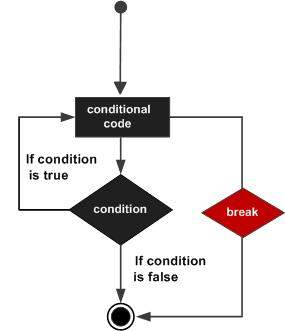# Python 3 - break statement

The break statement is used for premature termination of the current loop. After abandoning the loop, execution at the next statement is resumed, just like the traditional break statement in C.

The most common use of break is when some external condition is triggered requiring a hasty exit from a loop. The break statement can be used in both while and for loops.

If you are using nested loops, the break statement stops the execution of the innermost loop and starts executing the next line of the code after the block.

## Syntax

The syntax for a break statement in Python is as follows −

```break
```

## Flow Diagram## Example

```#!/usr/bin/python3

for letter in 'Python':     # First Example
if letter == 'h':
break
print ('Current Letter :', letter)

var = 10                    # Second Example
while var > 0:
print ('Current variable value :', var)
var = var -1
if var == 5:
break

print ("Good bye!")
```

## Output

When the above code is executed, it produces the following result −

```Current Letter : P
Current Letter : y
Current Letter : t
Current variable value : 10
Current variable value : 9
Current variable value : 8
Current variable value : 7
Current variable value : 6
Good bye!
```

The following program demonstrates use of the break in a for loop iterating over a list. User inputs a number, which is searched in the list. If it is found, then the loop terminates with the 'found' message.

## Example

```#!/usr/bin/python3

no = int(input('any number: '))
numbers = [11,33,55,39,55,75,37,21,23,41,13]

for num in numbers:
if num == no:
print ('number found in list')
break
else:
```

## Output

The above program will produce the following output −

```any number: 33
number found in list

any number: 5